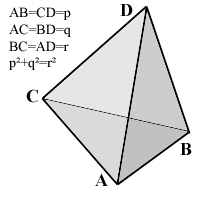All about flooble | fun stuff | Get a free chatterbox | Free JavaScript | Avatarsperplexus dot infoPythagorean Pyramid (Posted on 2004-04-26)The pictured tetrahedron has four identical rectangular (i.e., right-angled or pythagorean) triangles as faces, with

AB=CD=p,
AC=BD=q,
and p˛+q˛=r˛.

What's its volume, as a function of p, q and r?

 See The Solution Submitted by Federico Kereki Rating: 4.1250 (8 votes)Comments: ( Back to comment list | You must be logged in to post comments.)Slaying the Hedra (Spoiler) Comment 15 of 15 |Given p^2 + q^2 = r^2, the Pythagorean equation for a right triangle in Euclidean geometry, it can be seen that the shape is NOT a three-dimensional tetrahedron but a two-dimensional tetragon with parallel sides of lengths p and q (thus a paralleogram) with polygon diagonals of length r.  Thus the shape has no volume but area only.
The AREA of the TETRAGON is then pq.

 Posted by Dej Mar on 2007-12-06 11:17:48Please log in:

 Search: Search body:
Forums (0)